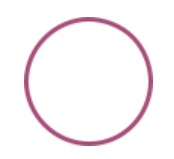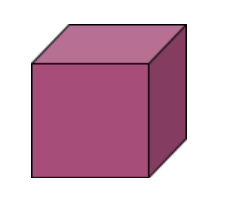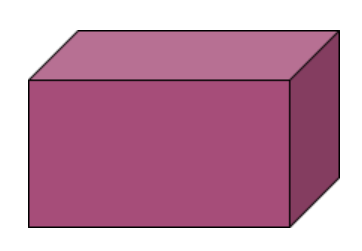Checkout JEE MAINS 2022 Question Paper Analysis : Checkout JEE MAINS 2022 Question Paper Analysis :

# Class 8 Maths Chapter 10 Visualising Solid Shapes MCQs

Class 8 Maths Chapter 10 (Visualising Solid Shapes) MCQs are available online with solved answers. The MCQs are as per the new exam pattern. These objective questions are formulated by experts, according to the latest CBSE syllabus (2021-2022) and NCERT curriculum. The chapter-wise multiple-choice questions are given at BYJU’S to make students learn each topic and help them to score good marks in exams.

Also, learn important questions for class 8 Maths here.

Practice more and test your skills on Class 8 Maths Chapter 10 Visualising Solid Shapes MCQs with the given PDF here.

## MCQs Questions on Class 8 Visualising Solid Shapes

Multiple choice questions (MCQs) are given for the Class 8 Visualising Solid Shapes chapter. All questions here will be provided with four multiple choices, among which one is the right answer.

1. A cube has ______ faces.

A. 4

B. 5

C. 6

D. 8

Explanation: A cube is a three-dimensional shape having six faces.

2. Number of vertices a cone has:

A. One

B. Two

C. Three

D. Four

3. A sphere has how many faces?

A. One

B. Two

C. Four

D. None

Explanation: A sphere is a round-shaped object having no faces and edges.

4. An example of cone is:

A. Tube

B. A tent

C. A ball

D. A box

5. The top-view of a cone looks like this:

A. A Circle

B. A Square

C. A Rectangle

D. A Triangle

Explanation: The base of a right cone is always circular. When we see it from the top, it looks like a circle with its vertex as the center of the circle.

6. The top-view of a cube looks like a:

A. Circle

B. Square

C. Rectangle

D. Triangle

Explanation: Since all the faces of a cube are of equal length.

7. The top-view of a cuboid looks like a:

A. Circle

B. Square

C. Rectangle

D. Triangle

8. The side-view of a cone appears as:

A. Circle

B. Square

C. Rectangle

D. Triangle

9. The base of a prism is:

A. Circle

B. Triangle

C. Square

D. Any shape

Explanation: A pyramid is a polyhedron whose base is a polygon (of any number of sides) and whose lateral faces are triangles with a common vertex.

10. If a polyhedron has 6 vertices and 12 edges. What is the number of faces it has?

A. 6

B. 8

C. 12

D. 18

Explanation: By Euler’s formula we know that:

For any polyhedron,

F + V – E = 2

F = 2-V+E

F = 2 – 6+12 = 8

11. The example of cylindrical shape is:

A. Pipes

B. Gas cylinder

C. Cold drink cans

D. All of the above

Answer: D. All of the above

12. What is the name of the shape?A. Circle

B. Square

C. Cone

D. Cylinder

13. What is the name of the given shape?A. Square

B. Rectangle

C. Cuboid

D. Cube

14. Find the name of the given shape.A. Square

B. Rectangle

C. Cuboid

D. Cube

15. A polyhedron is said to be regular if its faces are made up of regular polygons and the same number of faces meet at each vertex.

A. True

B. False

16. Prisms and Pyramids are:

A. Polygons

B. Cubes

C. Polyhedrons

D. Spherical shapes

17. A square is a 3D shape.

A. True

B. False

A square is a 2D Shape.

18. A cube is a 3D shape.

A. True

B. False

19. In the map, symbols are used to depict the different objects/places.

A. True

B. False